Goseeko blog# What is the ratio test?

## Overview

The ratio test is also known as D Alembert’s ratio test. It was first published by Jean le Rond d’ Alembert.

It is sometimes called the Cauchy ratio test. This test is the criterion for the convergence of a series.

By using this test we check the convergence of any series. If the limit of the ratio between consecutive terms is less than 1 then we can say that the series is divergent.

## Definition of sequence

A sequence is a function from the domain set of natural numbers N to any set S.

The real sequence is a function from N to R, the set of real numbers; denoted by f: N → R. Thus the real sequence f is set of all ordered pairs {n, f (n)}|{n = 1, 2, 3, . . .} i.e., set of all pairs (n, f (n)) with n a positive integer.

Note- Infinite sequence is a sequence in which the number of terms is infinite

## Ratio test

Statement- suppose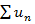is a series of positive terms  such that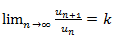then,

1. If k<1 , the series will be convergent.

2. If k>1 , then the series will be divergent.

3. The test fails when k = 1

## Solved examples

Example-1: Test the convergence of the series whose n’th term is given below-

n’th term =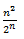sol.

We have

By D’Alembert ratio test,

So that by D’Alembert test, the series will be convergent.

Example: test the convergence of the following series whose nth term is

Sol:

Hence the ratio test fails.

Now using comparison test,

Since

Since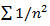is a harmonic series (with p = 2) which is convergent, so is the series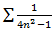Example: test the convergence of the following series whose nth term is

Solution:

So the series is convergent.

Interested in learning about similar topics? Here are a few hand-picked blogs for you!